# Python 中的图像增强技术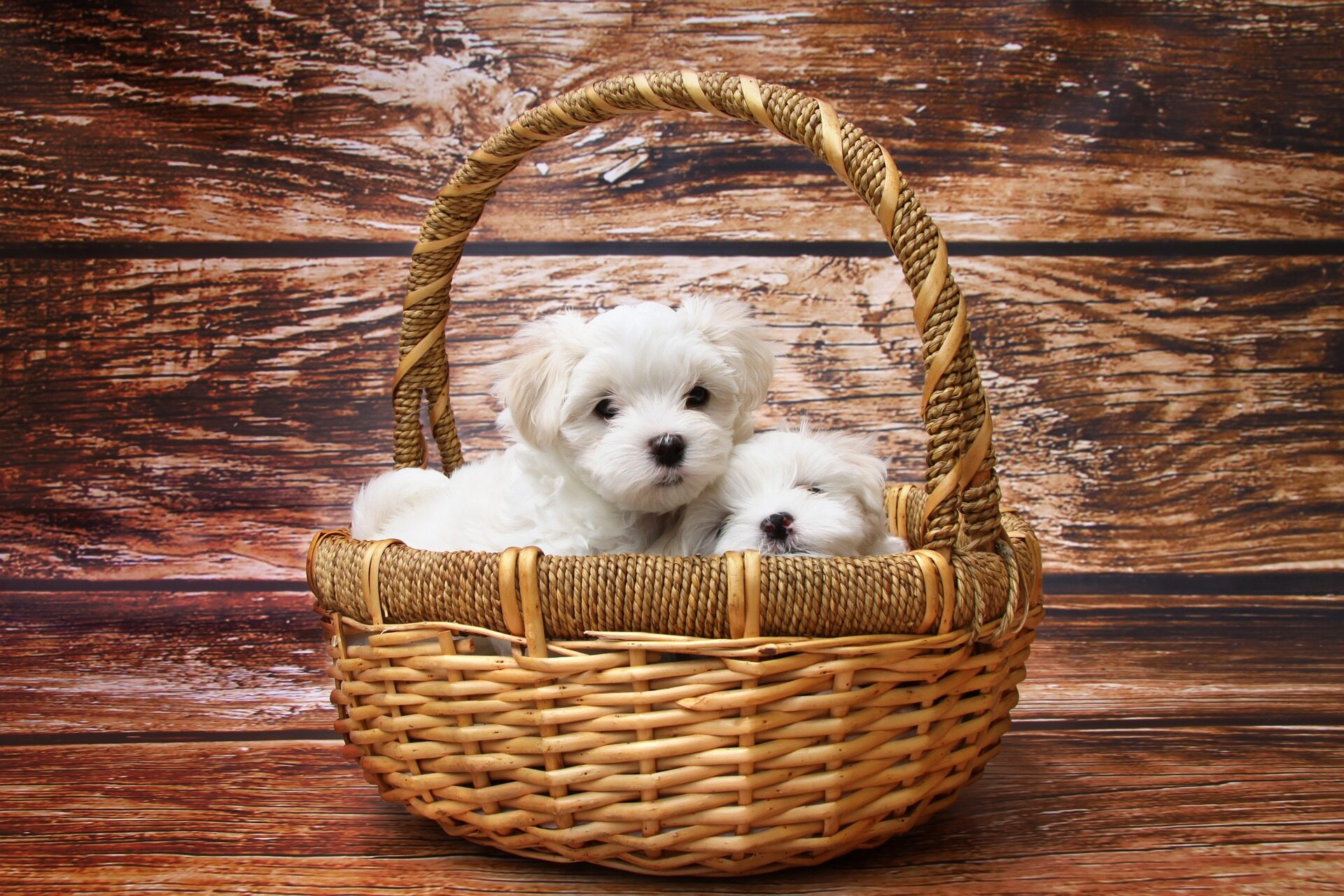• 离线或预处理增强

• 在线或实时增强

## 基本图像处理技术

• 翻转：垂直或水平翻转图像
• 旋转：将图像旋转指定的角度。
• 剪切：像平行四边形一样移动图像的一部分
• 裁剪：对象以不同比例出现在图像中的不同位置
• 放大，缩小
• 改变亮度或对比度

## imgaug

imgaug 是一个用于图像增强以及关键点 / 地标，边界框，热图和分段图的库。

pip install imgaug

pip install imgaug — upgrade — no-deps

### 导入所需的库

import imageioimport imgaug as iaimport imgaug.augmenters as iaaimport numpy as npimport pandas as pdimport matplotlib.pyplot as pltimport matplotlib.patches as patchesimport matplotlib%matplotlib inline

### 显示原始图像

image = imageio.imread(“.\\car2.jpeg”)ia.imshow(image)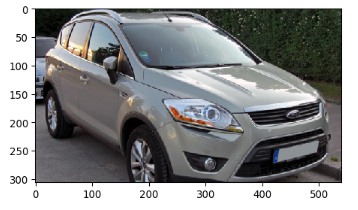### 旋转影像

rotate=iaa.Affine(rotate=(-50, 30))rotated_image=rotate.augment_image(image)ia.imshow(rotated_image)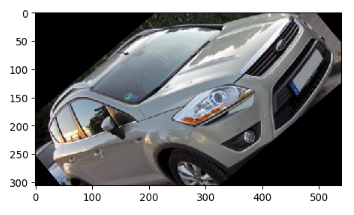### 给图像添加噪点

gaussian_noise=iaa.AdditiveGaussianNoise(10,20)noise_image=gaussian_noise.augment_image(image)ia.imshow(noise_image)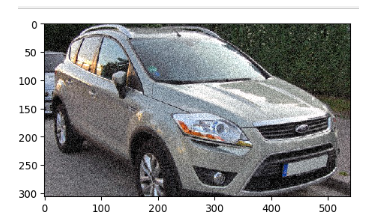### 裁剪图像

crop = iaa.Crop(percent=(0, 0.3)) # crop imagecorp_image=crop.augment_image(image)ia.imshow(corp_image)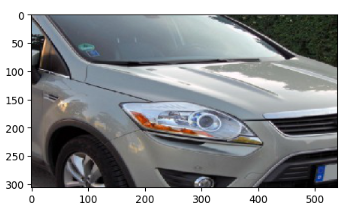### 扭曲图像

shear = iaa.Affine(shear=(0,40))shear_image=shear.augment_image(image)ia.imshow(shear_image)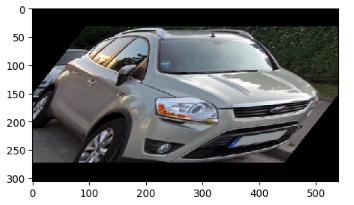### 翻转图像

#flipping image horizontallyflip_hr=iaa.Fliplr(p=1.0)flip_hr_image= flip_hr.augment_image(image)ia.imshow(flip_hr_image)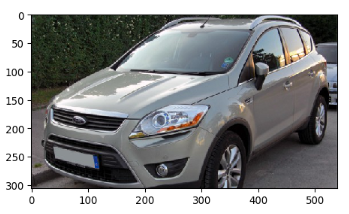### 垂直翻转图像

flip_vr=iaa.Flipud(p=1.0)flip_vr_image= flip_vr.augment_image(image)ia.imshow(flip_vr_image)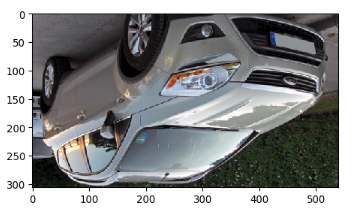### 改变图像的亮度

image = imageio.imread(“.\\img Aug\\car2.jpeg”)contrast=iaa.GammaContrast(gamma=2.0)contrast_image =contrast.augment_image(image)ia.imshow(contrast_image)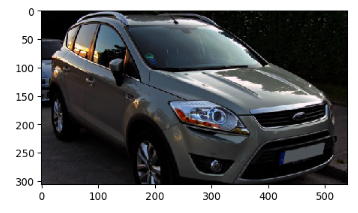### 缩放图像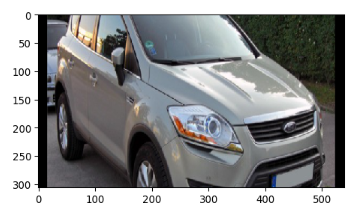### 增强物体检测

imgaug 支持边界框。 当我们旋转，剪切或裁剪图像时，对象周围的边界框也会相应更新。

from imgaug.augmentables.bbs import BoundingBox, BoundingBoxesOnImage

bbs = BoundingBoxesOnImage([ BoundingBox(x1=10, x2=520, y1=10, y2=300)], shape=image.shape)

ia.imshow(bbs.draw_on_image(image, size=2))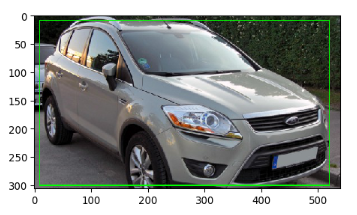move=iaa.Affine(translate_percent={"x": 0.1}, scale=0.8)image_aug, bbs_aug = move(image=image, bounding_boxes=bbs)ia.imshow(bbs_aug.draw_on_image(image_aug, size=2))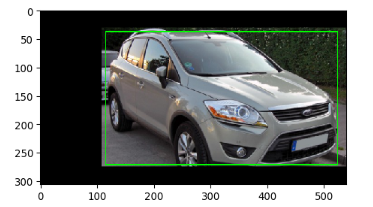### 应用图像增强后在图像外部处理边界框

rotate_bb=iaa.Affine(rotate=(-50, 30))image_aug, bbs_aug = rotate_bb(image=image, bounding_boxes=bbs)ia.imshow(bbs_aug.draw_on_image(image_aug, size=2))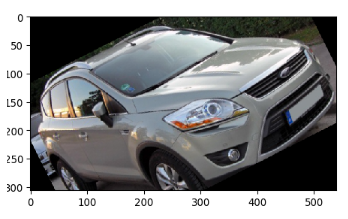• 将边框完全或部分移出图像
• 裁剪部分位于外部的边界框，使其完全位于图像内部

def pad(image, by):    image_border1 = ia.pad(image, top=1, right=1, bottom=1, left=1,                           mode="constant", cval=255)    image_border2 = ia.pad(image_border1, top=by-1, right=by-1,                           bottom=by-1, left=by-1,                           mode="constant", cval=0)    return image_border2

def draw_bbs(image, bbs, border):    GREEN = [0, 255, 0]    ORANGE = [255, 140, 0]    RED = [255, 0, 0]    image_border = pad(image, border)    for bb in bbs.bounding_boxes:        if bb.is_fully_within_image(image.shape):            color = GREEN        elif bb.is_partly_within_image(image.shape):            color = ORANGE        else:            color = RED        image_border = bb.shift(left=border, top=border)\                         .draw_on_image(image_border, size=2, color=color)return image_border

rotate=iaa.Affine(rotate=(-50, 30))image_aug, bbs_aug = rotate(image=image, bounding_boxes=bbs)image_after = draw_bbs(image_aug, bbs_aug.remove_out_of_image().clip_out_of_image(), 100)ia.imshow(image_after)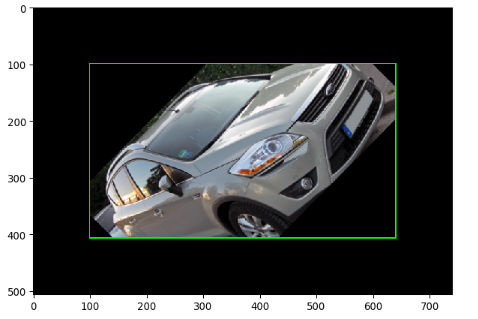https://towardsdatascience.com/data-augmentation-techniques-in-python-f216ef5eed69